# 1 2021-11-22

Tags: #untagged

Refs: ?

## 1.1 01:01

• You can define $$p{\hbox{-}}$$curvature for arithmetic schemes. Are there analogs of the Riemann curvature tensor? Ricci curvature?
• How to make Floer or Morse homology work for varieties or schemes? Not clear how to define things like gradient flows algebraically.

## 1.2 UGA Topology Seminar, Irving Dai, Equivariant Concordance and Knot Floer Homology

• WIP! Joint with Mallick and Stoffregen.
• Equivariant knots: pairs $$(K, \tau)$$ where $$K \subseteq S^3$$ and $$\tau: S^3{\circlearrowleft}$$ an orientation-preserving involution preserving $$K$$, so $$\tau(K) = K$$.
• Symmetries of the trefoil: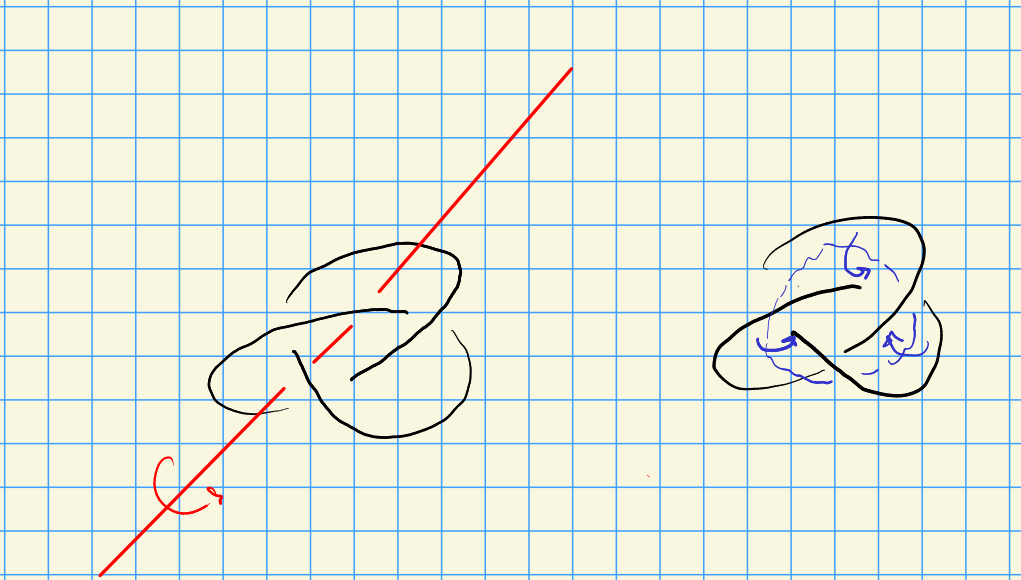• The first case is a strong inversion, the second is a 2-periodic involution (given by twisting about a core torus).

• One can assume that $$\tau$$ is rotation about some axis.

• There is an extension of $$\tau$$ to $${\mathbb{B}}^4$$, so define an equivariant slice surface $$\Sigma$$ if $$\tau \Sigma = \Sigma$$, and define an equivariant (slice?) genus as the minimal genus among such surfaces $$\tilde g_4(K)$$

• Study $$\tilde g_4(K) - g_4(K)$$. Boyle-Issan show this difference is unbounded for a family of periodic knots.

• Prove a similar theorem: given $$(K, \tau)$$, define a set of numerical invariants using Floer homology which are

• Equivariant concordance invariants
• Functions of these bound $$\tilde g_4(K)$$ from below.
• Produced a family of strongly invertible slice knots where $$\tilde g_4$$ is unbounded.

• Most (small crossing) knots admit a strong inversion.

• Next: how to apply this machinery to seemingly non-equivariant things.

• A slice surface $$\Sigma$$ is isotopy equivariant iff $$\tau_{{\mathbb{B}}^4} \isotopic \Sigma$$ rel boundary. Define isotopy equivariant genus $$\tilde{ig}_4(K)$$ as the minimal genus of such $$\Sigma$$.

• Calculating this invariants gives a way of finding non-isotopic surfaces for $$K$$.

• Recent work: topologically isotopic but not smoothly isotopic surfaces.

• JMZ: higher genus construction using knot floer homology. Needs high genus, won’t work for slice discs.
• H, HS: for slice discs using Khovanov homology.
• Proving topologically isotopic: a known theorem involving equivalence of $$\pi_1$$.

• Theorem: produced a knot where $$\tilde{ig}_4(K) > 0$$.

• Does $${\mathbb{B}}^4$$ actually matter here? The answer is no, can take $$\operatorname{ZHB}^4$$.

• A generalized isotopy equivariant surface is a triple $$(W, \tau_W, \Sigma)$$ where

• $$W \in \operatorname{ZHB}^4$$ with $${{\partial}}W = S^3$$
• $$\tau_W: W\to W$$ extends $$\tau$$ (in any way!)
• $$\tau_W \Sigma \isotopic \Sigma$$ rel $$K$$.
• Another application: let $$\Sigma, \Sigma'$$ be two slices surfaces in $${\mathbb{B}}^4$$ for $$K$$. Interpolate: take $$\Sigma = \Sigma_0 \to \Sigma_1 \to \cdots \to \Sigma_n = \Sigma'$$ where each arrow is a stabilization or destabilization or isotopy rel $$K$$. How many arrows are needed? Define this as $$M_{{~\mathrel{\Big\vert}~}}(\Sigma, \Sigma')$$, the stabilization number.

• Q: given a number of arrows, can this be achieved by picking a suitable genus?
• Theorem: for any $$m$$, produce a knot $$J_m$$ with two slice disks with stabilization distance exactly $$m$$.

• Theorem: if $$(K, \tau)$$ is strongly invertible slice and $$\Sigma$$ is any slice disk for $$K$$, then $$M_{{~\mathrel{\Big\vert}~}}(\Sigma, \tau_{{\mathbb{B}}^4} \Sigma) \geq \cdots$$, some function of the numerical invariants.

• So this can show non-isotopic, and require many stabilizations to become isotopic.
• These all induce maps on $$\CFK(K)$$, where the $$\tau$$ action induces a $$\tau$$ action on $$\CFK(K)$$. Isotopy equivariant knot cobordisms $$K_1\to K_2$$ induce $$\tau{\hbox{-}}$$equivariant maps $$\CFK(K_1) \to \CFK(K_2)$$ in the sense that this commutes with the two different $$\tau$$ actions on either side.

• Can use this to find knots that are concordant but not equivariantly concordant by using algebraic restrictions on bigraded $$\CFK(K)$$

• Doing this with higher order diffeomorphisms: the roadblock is defining $$\operatorname{HF}$$ mod $$p$$!

## 1.3 19:57

A nice modern intro to homotopy theory: https://www.uni-regensburg.de/Fakultaeten/nat_Fak_I/Bunke/intro-homoto.pdf

Quotients are colimits: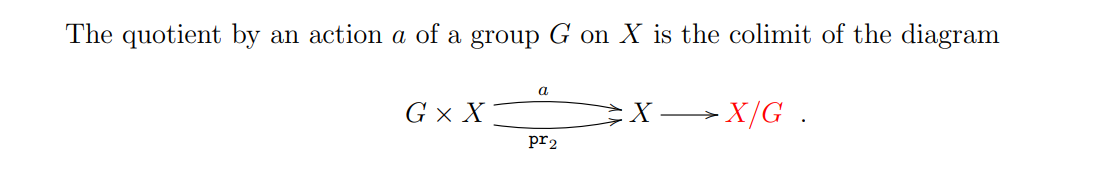Geometric realization as a \begin{align*}\[coend\end{align*} ]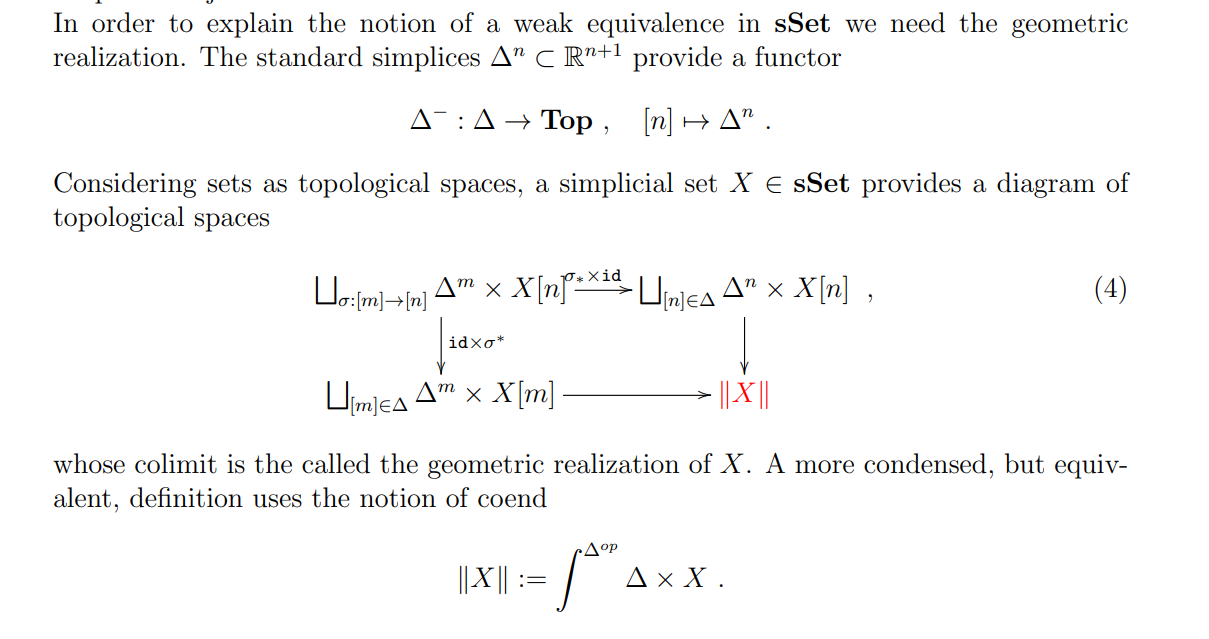Homotopy fibers: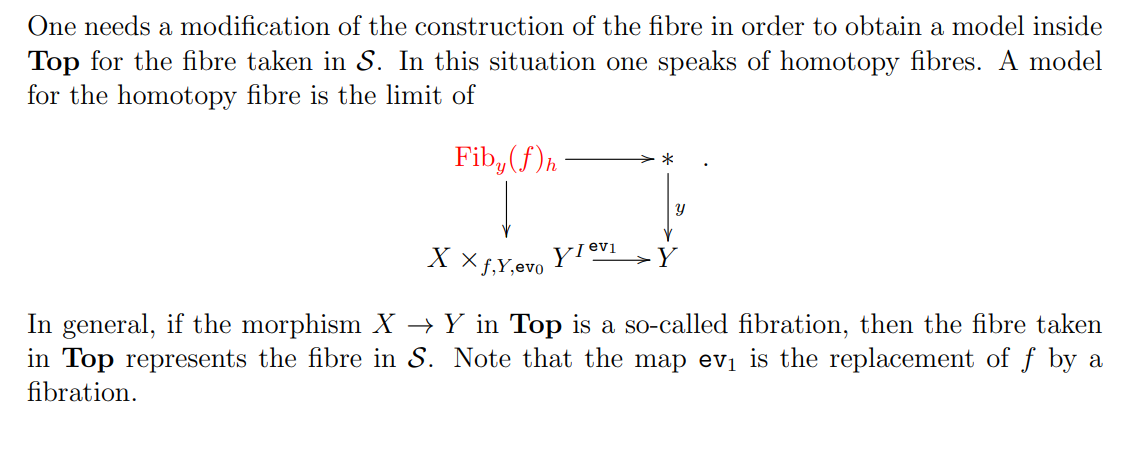Homotopy cofiber: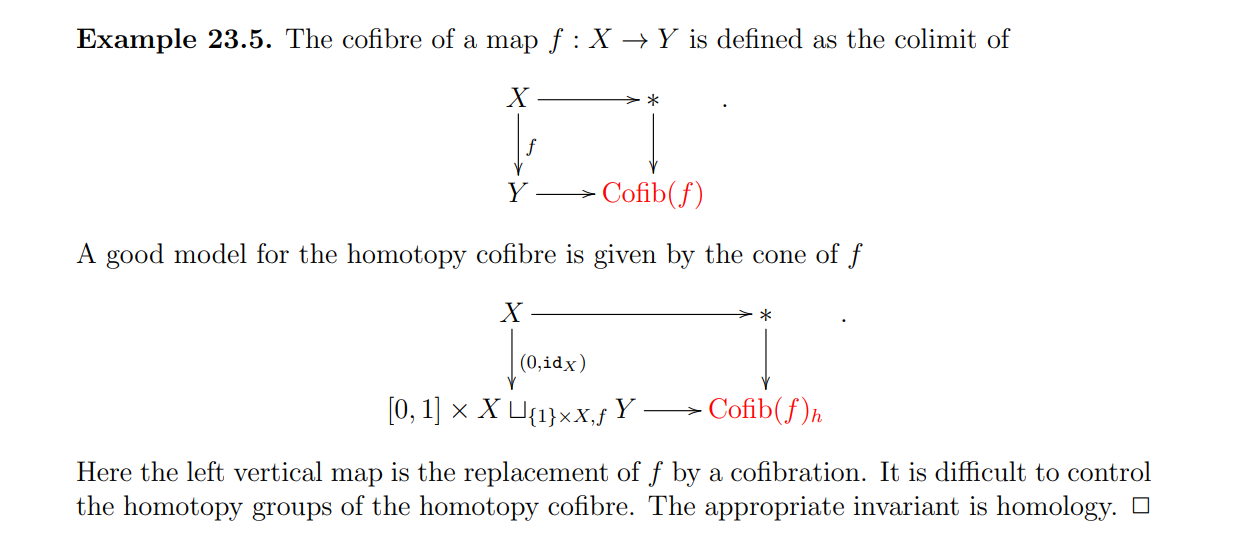Spectra as a presentable \begin{align*}\[infty-category\end{align*} ]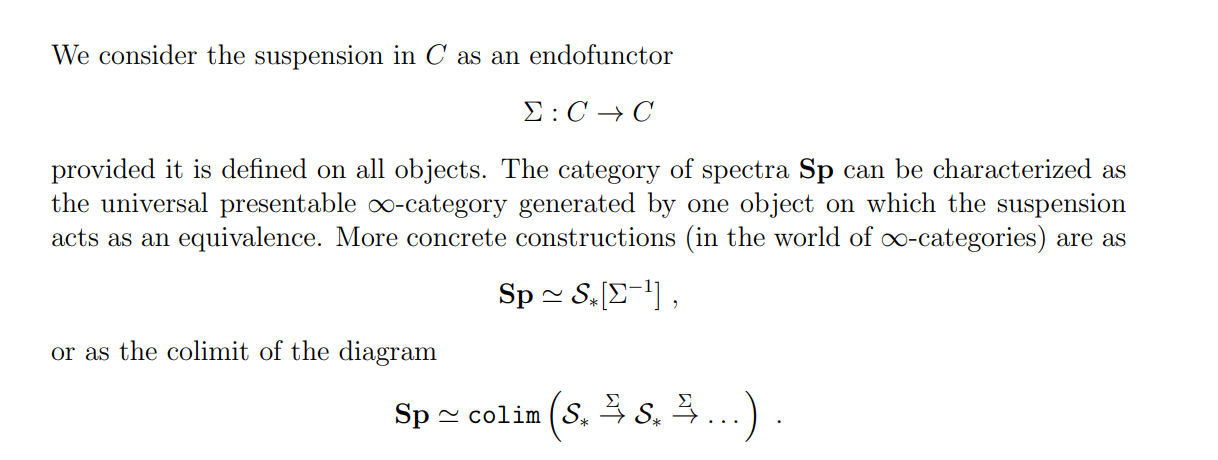# 2 2021-11-10

Tags: #untagged

Refs: ?

## 2.1 16:20

Hector Pasten, UGA NT seminar.

• Mordell’s conjecture: for $$C$$ a curve, $$C({\mathbb{Q}}) < \infty$$.
• Chabauty: if $${\operatorname{rank}}J({\mathbb{Q}}) > g = \dim J({\mathbb{Q}})$$, then $$C({\mathbb{Q}})$$ is finite.
• Faltings: Proof using heights on moduli spaces
• Vojta: Proof by Diophantine approximation.
• Abel-Jacobi map: $$C\to J_C$$ by $$x \mapsto [x-x_0]$$.
• Chabauty’s proof: let $$\Gamma$$ be the $$p{\hbox{-}}$$adic closure of $$J({\mathbb{Q}})$$ in $$J({ {\mathbb{Q}}_p })$$, which is a $$p{\hbox{-}}$$adic Lie subgroup of $$J({ {\mathbb{Q}}_p })$$. Interpret $$\Gamma \cap C({ {\mathbb{Q}}_p })$$ as zero loci of $$p{\hbox{-}}$$adic analytic functions of $$C({ {\mathbb{Q}}_p })$$, constructed using integration.
• See \begin{align*}\[good reduction\end{align*} ], \begin{align*}\[hyperplane section\end{align*} ].
• Nice: smooth, projective, \begin{align*}\[geometrically irreducible\end{align*} ].
• Looks hyperbolic: contains no elliptic curves.
• First Chern number: self-intersection of the canonical divisor.
• Reduction map $${ \text{red} }: A({ {\mathbb{Q}}_p }) \to A({\mathbb{F}}_p)$$, take residue discs $$U_x \mathrel{\vcenter{:}}={ \text{red} }^{-1}(x)$$ for $$x\in A({\mathbb{F}}_p)$$. Bound the number of points in $$X({\mathbb{Q}}_p) \cap\Gamma \cap U_x$$.
• Fat point: $${ {\mathbb{Q}}_p }[z]/\left\langle{z^n}\right\rangle$$ for some $$n> 1$$.

# 3 2021-11-09

Tags: #untagged

Refs: ?

## 3.1 00:11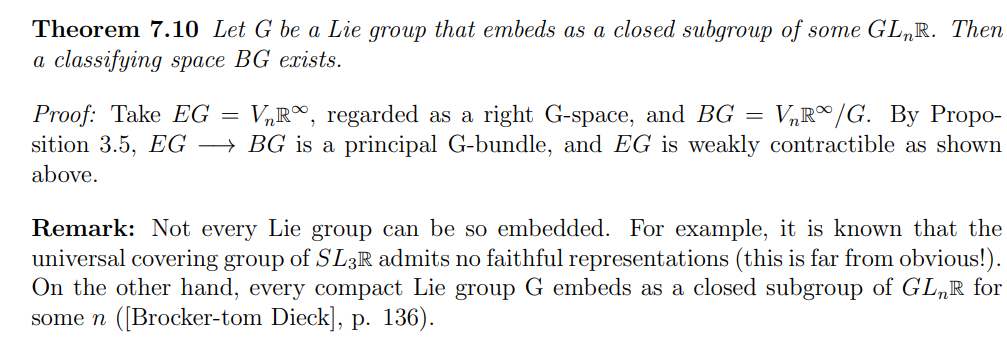## 3.2 15:51

• Torelli: the map sending a curve to its Jacobian is an injection on points.

• Intermediate Jacobian: introduce to prove irrationality of cubic threefolds. An abelian variety the parameterizes degree zero cycles in dimension 1, up to rational equivalence.

• The pair $$(J(X), \Theta)$$ determines a cubic threefold, where $$\Theta$$ is the theta divisor, which has a unique singular point.
• Relationship between complex projective and geometry and symplectic topology: Kähler manifolds.

• Abouzaid: interesting results about symplectic topology of Hamiltonian fibrations over the 2-sphere, and their consequences for smooth projective maps over the projective line.

• The Grothendieck group of mixed Hodge modules, which enhances the Grothendieck group of $$G{\hbox{-}}$$modules.

• A motivic semiorthogonal decomposition is the decomposition of the derived category of a quotient stack \begin{align*}X/G\end{align*} into components related to the “fixed-point data.” They represent a categorical analog of the Atiyah-Bott localization formula in equivariant cohomology, and their existence is conjectured for finite G

• Can define curvature and 2nd fundamental form for algebraic varieties?

• Invariants like HOMFLY: invariants of quantum matrices

• consider the stack of representations, its inertia stack and the nilpotent version of the inertia stack.

• Hurwitz spaces H_{k,g}, parametrizing degree k, genus g covers of P^1

• Kobayashi–Hitchin correspondence, which states that a holomorphic vector bundle on a compact Kähler manifold admits a Hermite–Einstein metric if and only if the bundle is slope polystable

• predicted that given two vector bundles V_1, V_2 whose first Chern classes both vanish and whose second Chern classes agree, the resulting line bundles Thom(V_1) and Thom(V_2) should agree in Pic(Ell_G(X)).

# 4 2021-11-08

Tags: #untagged

Refs: ?

## 4.1 15:05

Hannah Turner, GT: Branched Cyclic Covers and L-Spaces

• Two main constructions for 3-manifolds: Dehn surgery and branched cyclic covers

• Idea: $$C_n\curvearrowright M$$, take quotient to get an $$n{\hbox{-}}$$fold covering map away from a branch locus (usually a knot or link).

• Given a knot $$K\hookrightarrow S^3$$, can produce a canonical cyclic branched cover for any $$n$$, $$\Sigma_n(K)$$.

• Dehn surgeries: classified by $$p/q \in {\mathbb{Q}}$$.

• Fact: $$\dim_{{\mathbb{F}}_2} \widehat{\operatorname{HF}}(M) \geq \# H_1(M; {\mathbb{Z}})$$ unless it’s infinite, in which case we set the RHS to zero. We say $$M$$ is an $$L{\hbox{-}}$$space if this is an equality.

• Note that lens spaces have this property!
• Conjecture: non $$L{\hbox{-}}$$space if and only if admits a co-oriented taut foliation (decomposition into surfaces) iff $$\pi_1$$ is left orderable.

Q: push through local system correspondence, what does this say about reps $$\pi_1\to G$$..? Or local systems..?

• We know foliation $$\implies$$ non $$L{\hbox{-}}$$space, the other directions are all wide open.

• Diagrams for knots: boxes with numbers are half-twists, sign prescribes directions.

• Which branched covers of knots are $$L{\hbox{-}}$$spaces?

• Nice trick: quotient by a $$C_2$$ action to make it a double branched cover $$X\to X/C_2$$, and find an $$n{\hbox{-}}$$fold branched cover $$\tilde X\to X$$. Then take an $$n{\hbox{-}}$$fold branched cover $$\tilde{X/C_2} \to X/C_2$$ and then its 2-fold branched cover will be $$\tilde X \to \tilde{X/C_2}$$.

• Weakly quasi-alternating $$K$$: $$\Sigma_2(K)$$ is an $$L{\hbox{-}}$$space.

• There are tools for showing the $$\pi_1$$ you get here are not left-orderable. Showing left-orderability: fewer tools, need representation theory.

• Generalized $$L{\hbox{-}}$$space: $$L{\hbox{-}}$$spaces for $$S^1\times S^2$$.

# 5 2021-11-05

Tags: #untagged

Refs: ?

## 5.1 01:31

Differential forms on (derived) \begin{align*}\[stacks\end{align*} ]: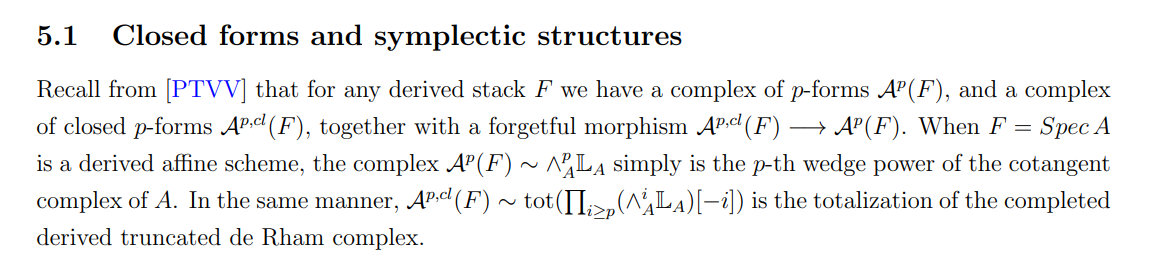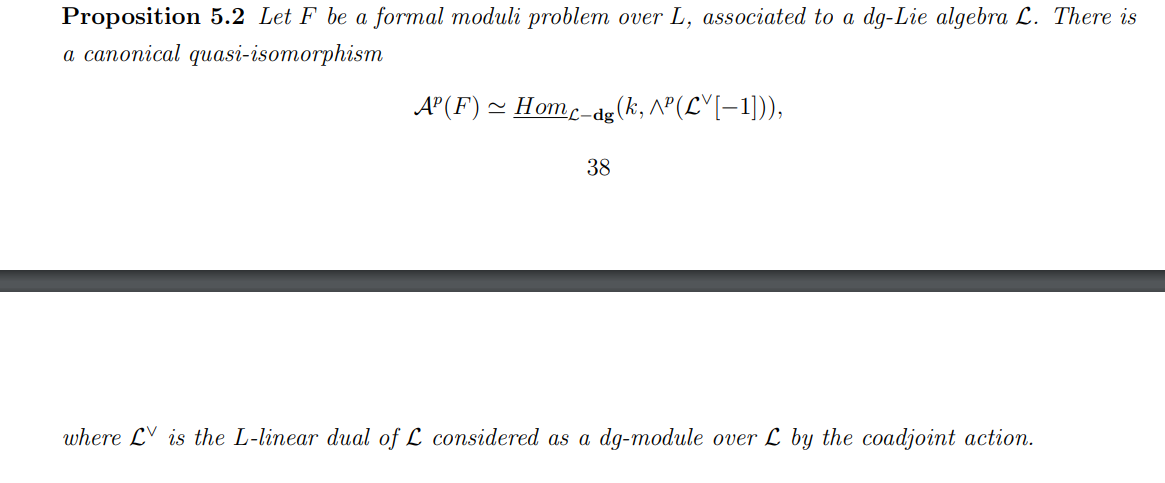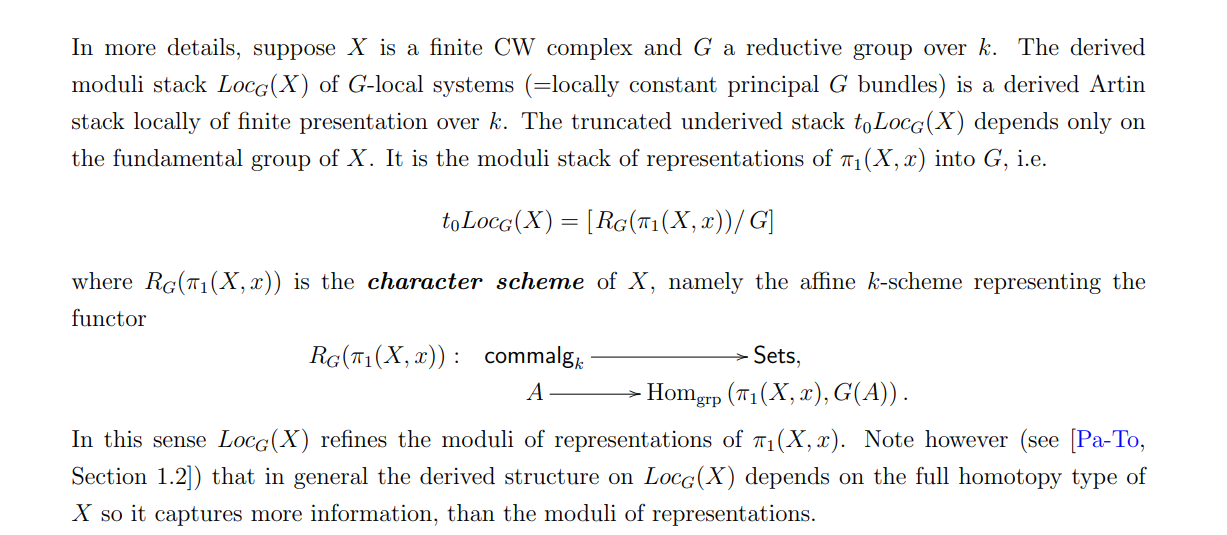What is non-commutative geometry?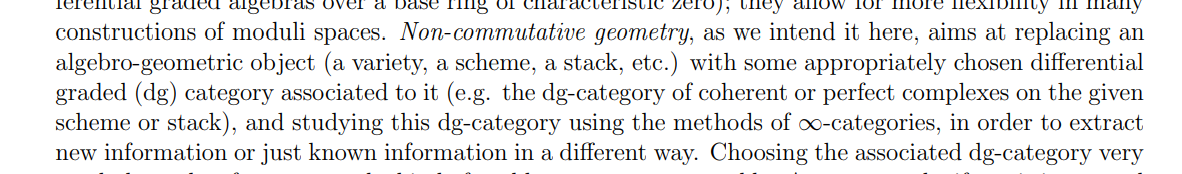Category of singularities: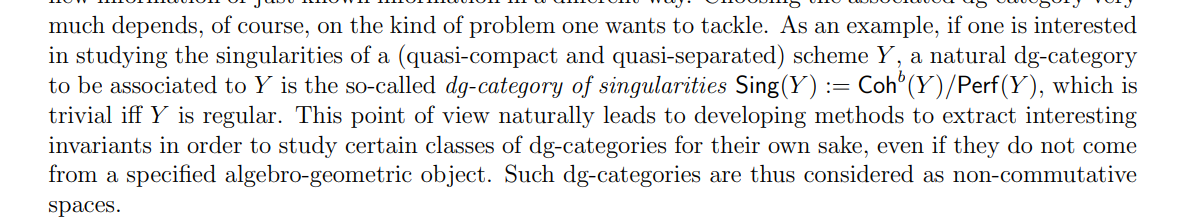Bloch’s conductor conjecture: